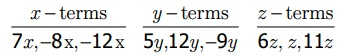Home | | Maths 7th Std | Exercise 3.1

# Exercise 3.1

7th Maths : Term 1 Unit 3 : Algebra : Exercise 3.1 : Fill in the blanks, Say true or false, Find the value of the following, Objective type questions, Text Book Back Exercises Questions with Answers, Solution

Exercise 3.1

1. Fill in the blanks:

(i) The variable in the expression 16x − 7 is x.

(ii) The constant term of the expression 2y − 6 is –6.

(iii) In the expression 25m + 14n, the type of the terms are variable terms.

(iv) The number of terms in the expression 3ab + 4c –9 is three.

(v) The numerical co-efficient of the term −xy is – 1.

2. Say True or False.

(i) x + ( −x) = 0 (True)

(ii) The co-efficient of ab in the term 15abc is 15. (False)

(iii) 2pq and − 7qp are like terms (True)

(iv) When y = −1, the value of the expression 2y − 1 is 3 (False)

3. Find the numerical coefficient of each of the following terms: −3yx, 12k, y, 121bc, − x, 9pq, 2ab

–3yx, 12k, y, 121bc, – x, 9pq, 2ab

The numerical coefficients –3, 12, 1, 121, –1, 9, 2

4. Write the variables, constants and terms of the following expressions.

(i) 18 + xy (ii) 7p − 4q + 5 (iii) 29x + 13y (iv) b + 25. Identify the like terms among the following : 7x, 5y, −8x, 12y, 6z, z, −12x, −9y, 11z.

Like terms :

x terms : 7x, –8x, –12x

y terms : 5y, 12y, –9y

z terms : 6z, z, 11z6. If x = 2 and y = 3, then find the value of the following expressions

(i) 2x – 3y (ii) x + y (iii) 4yx (iv) x + 1 – y

x = 2, y = 3

i) 2x – 3y = 2(2) – 3(3)

= 4 – 9 = –5

ii) x + y = 2 + 3 = 5

iii) 4yx = 4 (3) – 2 = 12 – 2 = 10

iv) x + 1 – y = 2 + 1 – 3 = 3 – 3 = 0

Objective type questions

7. An algebraic statement which is equivalent to the verbal statement “Three times the sum of x and y” is

(i) 3(x + y)

(ii) 3 + x + y

(iii) 3x + y

(iv) 3 + xy

8. The numerical co-efficient of −7mn is

(i) 7

(ii) −7

(iii) p

(iv) −p

9. Choose the pair of like terms

(i) 7p, 7x

(ii) 7r, 7x

(iii) −4x, 4

(iv) −4x, 7x

10. The value of 7a − 4b when a = 3, b = 2 is

(i) 21

(ii) 13

(iii) 8

(iv) 32

EXERCISE-3.1

1. (i) x

(ii) −6

(iii) unlike

(iv) three

(v) −1

2. (i) True (ii) False (iii) True (iv) False

3. −3,12, 1,121, −1,9,2.

4.5.6. (i) –5 (ii) 5 (iii) 10 (iv) 0

Objective type questions

7. (i) 3(x+y)

8. (ii) –7

9. (iv) −4x , 7x

10. (ii) 13

Tags : Questions with Answers, Solution | Algebra | Term 1 Chapter 3 | 7th Maths , 7th Maths : Term 1 Unit 3 : Algebra
Study Material, Lecturing Notes, Assignment, Reference, Wiki description explanation, brief detail
7th Maths : Term 1 Unit 3 : Algebra : Exercise 3.1 | Questions with Answers, Solution | Algebra | Term 1 Chapter 3 | 7th Maths